Note: This is an archvied version of our old webpage. Some links might be broken. The current one can be found here.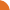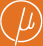Chair for Foundations of Software Reliability and Theoretical Computer Science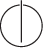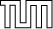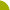Publications - Computing Quantiles in Markov Chains with Multi-Dimensional CostsReference: Christoph Haase, Stefan Kiefer, and Markus Lohrey. Computing quantiles in Markov chains with multi-dimensional costs. In Proceedings of the 32nd Annual Symposium on Logic in Computer Science (LICS). IEEE, 2017. Abstract: Probabilistic systems that accumulate quantities such as energy or cost are naturally modelled by cost chains, which are Markov chains whose transitions are labelled with a vector of numerical costs. Computing information on the probability distribution of the total accumulated cost is a fundamental problem in this model. In this paper, we study the so-called cost problem, which is to compute quantiles of the total cost, such as the median cost or the probability of large costs. While it is an open problem whether such probabilities are always computable or even rational, we present an algorithm that allows to approximate the probabilities with arbitrary precision. The algorithm is simple to state and implement, and exploits strong results from graph theory such as the so-called BEST theorem for efficiently computing the number of Eulerian circuits in a directed graph. Moreover, our algorithm enables us to show that a decision version of the cost problem lies in the counting hierarchy, a counting analogue to the polynomial-time hierarchy that contains the latter and is included in PSPACE. Finally, we demonstrate the applicability of our algorithm by evaluating it experimentally. Suggested BibTeX entry: @inproceedings{17HKL-LICS,     author = {Christoph Haase and Stefan Kiefer and Markus Lohrey},     booktitle = {Proceedings of the 32nd Annual Symposium on Logic in Computer Science (LICS)},     publisher = {IEEE},     title = {Computing Quantiles in {M}arkov Chains with Multi-Dimensional Costs},     year = {2017} }